# High School Math : Sectors

## Example Questions

1 2 4 Next →

### Example Question #17 : Sectors

What is the angle of a sector that has an arc length of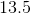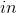on a circle of diameter?

Possible Answers: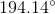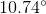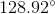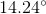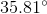Correct answer:Explanation:

The first thing to do for this problem is to compute the total circumference of the circle. Notice that you were given the diameter. The proper equation is therefore: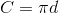For your data, this means,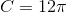Now, to compute the angle, note that you have a percentage of the total circumference, based upon your arc length: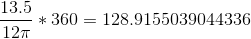Rounded to the nearest hundredth, this is.

1 2 4 Next →

### All High School Math Resources